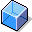ScalesDefinition

Freight depends on certain influential sizes, which are illustrated with scales. Scales are divided into levels that have different values.

A scale consists of:

• A scale header, which includes general information about the scale (scale base type, scale type, rounding rule)
• An unlimited amount of scale levels, each of which is made up of a value (such as weight, volume, postal code or distance) and a calculation type.

Each scale is an independent piece of master data that can be used in many different condition records. When you create a condition record, you can also maintain the condition rates or condition percentage rates according to the assigned scales.

Scale base type

The scale base type defines how the system interprets the scale. The scale can refer to weight or volume, for example. You can base a pricing element scale on one of the following criteria:

Examples of scale base types

• Gross weight
• Net weight
• Volume
• Distance
• Number of different means of transportation
• Time (travel time or wait time)
• Tariff zone (destination)
• Destination region

Scale type

A scale type is an indicator that controls how the scale levels are defined. The scale type indicates whether the scale values that were specified depict the upper- or lower boundaries for the scale levels.

• From a certain value, such as weight, onward (base scale)
• Up to a certain value, such as weight (to-scale)
• For exactly one value, such as postal code (same-scale)

Use

You can define scales when you create condition records. The scale you use determines how shipment costs are calculated. For example, you can use a scale to define that the freight rate that is used for calculation depends on the weight being transported and the distance it is being transported.

Freight rates can be maintained depending on scaling:

• Individual value (no scaling)
• One scale (weight, for example)

Maintain freight rates for each scale value

• Two or three scales (weight and postal code, for example)

Maintain freight rates for various combinations of the scale valuesExample

Example of a freight matrix

 Kilometers / Tons Minimum value up to5 tons up to10 tons up to20 tons up to25 tons Maximum value 2000.00 3200.00 5200.00 6000.00 up to 100 km 250.00 20.35 18.55 15.20 12.75 up to 500 km 450.00 33.60 29.50 25.20 21.30 up to 1,000 km 800.00 43.50 37.30 33.20 28.50 (and so on) 850.00 1.50 2.50 3.20 3.50

Weight scale

Scale base type: gross weight

Scale type: to-scale

Distance scale

Scale base type: distance

Scale type: to-scale

Determining freight:

Up to a scale value of 100 km and 5 tons, the scale rate is 20.35. For a scale value of 5 tons and 100 km to 500 km, the scale rate is 33.60. At a scale value of 90 km and 800 kg, the freight rate would be 20.35. The freight is calculated as follows: 8 * 20.35 = 162.80. Since the minimum value is 250, however, the freight charged in this case is 250.